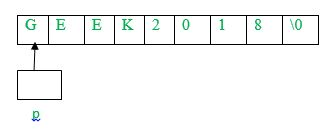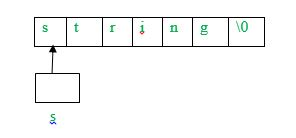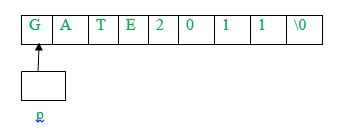# Practice questions on Strings

String is an important topic from GATE exam point of view. We will discuss key points on strings as well different types of questions based on that.

There are two ways to store strings as character array (char p) or a pointer pointing to a string (char* s = “string”), both of which can be accessed as arrays. Assuming p as character array and s as pointer pointing to a string, following are the key points:

• The operator sizeof(p) will give the memory allocated to character array. However, sizeof(s) will give size of pointer which is independent of the string pointed by p.
• Each string is appended by a null character (‘\0’) which depicts end of the string.
• The length of string can be calculated using strlen() function. However, the function does not include null character ‘\0’ in the length. For example, strlen(s) will return 6.
• Single character from strings can be printed as:
```printf(“%c”, s);
printf(“%c”, p);```
• Complete string can be printed as:
```printf(“%s”, s);
printf(“%s”, p);```
• As p is a character array, its individual elements can be modified (p[i] = ‘c’). However, using s (a pointer to string), individual elements of string can’t be modified.
• As s is a pointer, it can be pointed to any other string as well (s =”string2”). However, using p, it is not possible.

Let us discuss some problems based on the concepts discussed:

Que – 1. What does the following fragment of C-program print?

```char c[] = "GEEK2018";
char *p =c;
printf("%c,%c", *p,*(p+p-p));```

(A) G, 1
(B) G, K
(C) GEEK2018
(D) None of the above

Solution: As given in the question, p points to character array c[] which can be represented as:As p is a pointer of type character, *p will print ‘G’

Using pointer arithmetic,
*(p+p-p) = *(p+75-69) (Using ascii values of K and E) = *(p+6) = 1.
Therefore, the output will be G, 1.

Que – 2. Which of the following C code snippet is not valid?

(A) char* p = “string1”; printf(“%c”, *++p);
(B) char q[] = “string1”; printf(“%c”, *++q);
(C) char* r = “string1”; printf(“%c”, r);
(D) None of the above

Solution: Option (A) is valid as p is a pointer pointing to character ‘s’ of “string1”. Using ++p, p will point to character ‘t’ in “string1”. Therefore, *++p will print ‘t’.
Option (B) is invalid as q being base address of character array, ++q(increasing base address) is invalid.
Option (C) is valid as r is a pointer pointing to character ‘s’ of “string1”. Therefore,

`r = *(r+1) = ‘t’ and it will print ‘t’.`

Que – 3. Consider the following C program segment: (GATE CS 2004)

```char p;
char *s = "string";
int length = strlen(s);
int i;
for (i = 0; i < length; i++)
p[i] = s[length — i];
printf("%s",p);```

The output of the program is:
(A) gnirts
(B) gnirt
(C) string
(D) no output is printed

Solution: In the given code, p is declared as a character array and s is a pointer pointing to a string. The length will be initialized to 6. In the first iteration of for loop (i = 0),p[i] = s[6-0] and s is ‘\0’
Therefore, p becomes ‘\0’. As discussed, ‘\0’ means end of string. Therefore, nothing is printed as first character of string is ‘\0’.

Que - 4. What does the following fragment of C-program print?

```char c[] = "GATE2011";
char *p =c;
printf("%s", p + p - p) ;```

(A) GATE2011
(B) E2011
(C) 2011
(D) 011

Solution: As given in the question, p points to character array c[] which can be represented as:As p is a pointer of type character, using pointer arithmetic,
p + p - p = p + 69 - 65 (Using Ascii values of A and E) = p + 4

Now, p + 4 will point to 2, the string starting from 2 till ‘\0’ will be printed which is 2011.My Personal Notes arrow_drop_up

This article is contributed by Sonal Tuteja. If you like GeeksforGeeks and would like to contribute, you can also write an article using contribute.geeksforgeeks.org or mail your article to contribute@geeksforgeeks.org. See your article appearing on the GeeksforGeeks main page and help other Geeks.

Please Improve this article if you find anything incorrect by clicking on the "Improve Article" button below.

Article Tags :
Practice Tags :

4

Please write to us at contribute@geeksforgeeks.org to report any issue with the above content.